# Introduction

We will take a dive into the ‘College’ data set from the ISLR library in R and try to predict the number of applicants based on the other variables. The data set contains statistics for a large number of US Colleges from the 1995 issue of US News and World Report. There are 18 variables and 777 observations. This dataset was taken from the StatLib library which is maintained at Carnegie Mellon University. The dataset was used in the ASA Statistical Graphics Section’s 1995 Data Analysis Exposition.

Format: A data frame with 777 observations on 18 variables.

18 characteristics are reported:

[Private] Whether the college is public or private;

[Accept] Number of applications accepted;

[Enroll] Number of new students enrolled;

[Top10perc] Percentage of new students from top 10% of High School class

[Top25perc] Percentage new students from top 25% of High School class

[Outstate] Out-of-state tuition

[Room.Board] Room and board costs

[Books] Estimated book costs

[Personal] Estimated personal spending

[PhD] Percentage of faculty with Ph.D.’s

[Terminal] Percentage of faculty with terminal degree

[S.F.Ratio] Student/faculty ratio

[perc.alumni] Percentage alumni who donate

[Expend] Instructional expenditure per student

# Analysis

First, let us load the ISLR library.

library(ISLR)

Now let us get a summary of the variables within the College dataset.

summary(College)
##  Private        Apps           Accept          Enroll       Top10perc
##  No :212   Min.   :   81   Min.   :   72   Min.   :  35   Min.   : 1.00
##  Yes:565   1st Qu.:  776   1st Qu.:  604   1st Qu.: 242   1st Qu.:15.00
##            Median : 1558   Median : 1110   Median : 434   Median :23.00
##            Mean   : 3002   Mean   : 2019   Mean   : 780   Mean   :27.56
##            3rd Qu.: 3624   3rd Qu.: 2424   3rd Qu.: 902   3rd Qu.:35.00
##            Max.   :48094   Max.   :26330   Max.   :6392   Max.   :96.00
##  Min.   :  9.0   Min.   :  139   Min.   :    1.0   Min.   : 2340
##  1st Qu.: 41.0   1st Qu.:  992   1st Qu.:   95.0   1st Qu.: 7320
##  Median : 54.0   Median : 1707   Median :  353.0   Median : 9990
##  Mean   : 55.8   Mean   : 3700   Mean   :  855.3   Mean   :10441
##  3rd Qu.: 69.0   3rd Qu.: 4005   3rd Qu.:  967.0   3rd Qu.:12925
##  Max.   :100.0   Max.   :31643   Max.   :21836.0   Max.   :21700
##    Room.Board       Books           Personal         PhD
##  Min.   :1780   Min.   :  96.0   Min.   : 250   Min.   :  8.00
##  1st Qu.:3597   1st Qu.: 470.0   1st Qu.: 850   1st Qu.: 62.00
##  Median :4200   Median : 500.0   Median :1200   Median : 75.00
##  Mean   :4358   Mean   : 549.4   Mean   :1341   Mean   : 72.66
##  3rd Qu.:5050   3rd Qu.: 600.0   3rd Qu.:1700   3rd Qu.: 85.00
##  Max.   :8124   Max.   :2340.0   Max.   :6800   Max.   :103.00
##     Terminal       S.F.Ratio      perc.alumni        Expend
##  Min.   : 24.0   Min.   : 2.50   Min.   : 0.00   Min.   : 3186
##  1st Qu.: 71.0   1st Qu.:11.50   1st Qu.:13.00   1st Qu.: 6751
##  Median : 82.0   Median :13.60   Median :21.00   Median : 8377
##  Mean   : 79.7   Mean   :14.09   Mean   :22.74   Mean   : 9660
##  3rd Qu.: 92.0   3rd Qu.:16.50   3rd Qu.:31.00   3rd Qu.:10830
##  Max.   :100.0   Max.   :39.80   Max.   :64.00   Max.   :56233
##  Min.   : 10.00
##  1st Qu.: 53.00
##  Median : 65.00
##  Mean   : 65.46
##  3rd Qu.: 78.00
##  Max.   :118.00

How many pbservations have incomplete data?

sum(is.na(College))
##  0

Seems like there are no missing data.

pairs(College[,1:10])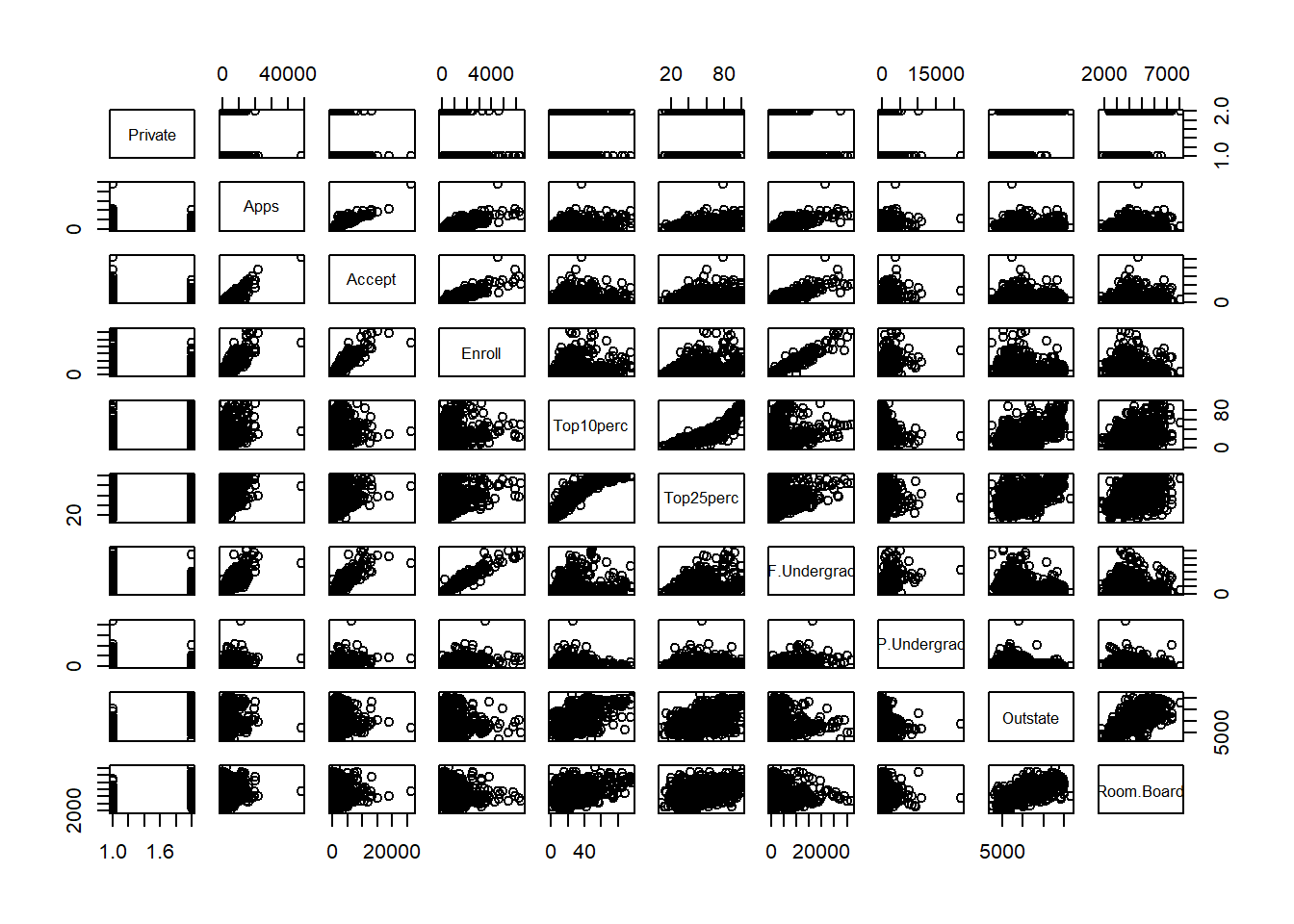We see that Apps has some positive correlation with Accept.

Now we will plot the remaining variables with Apps:

pairs(College[,c(2,11:18)])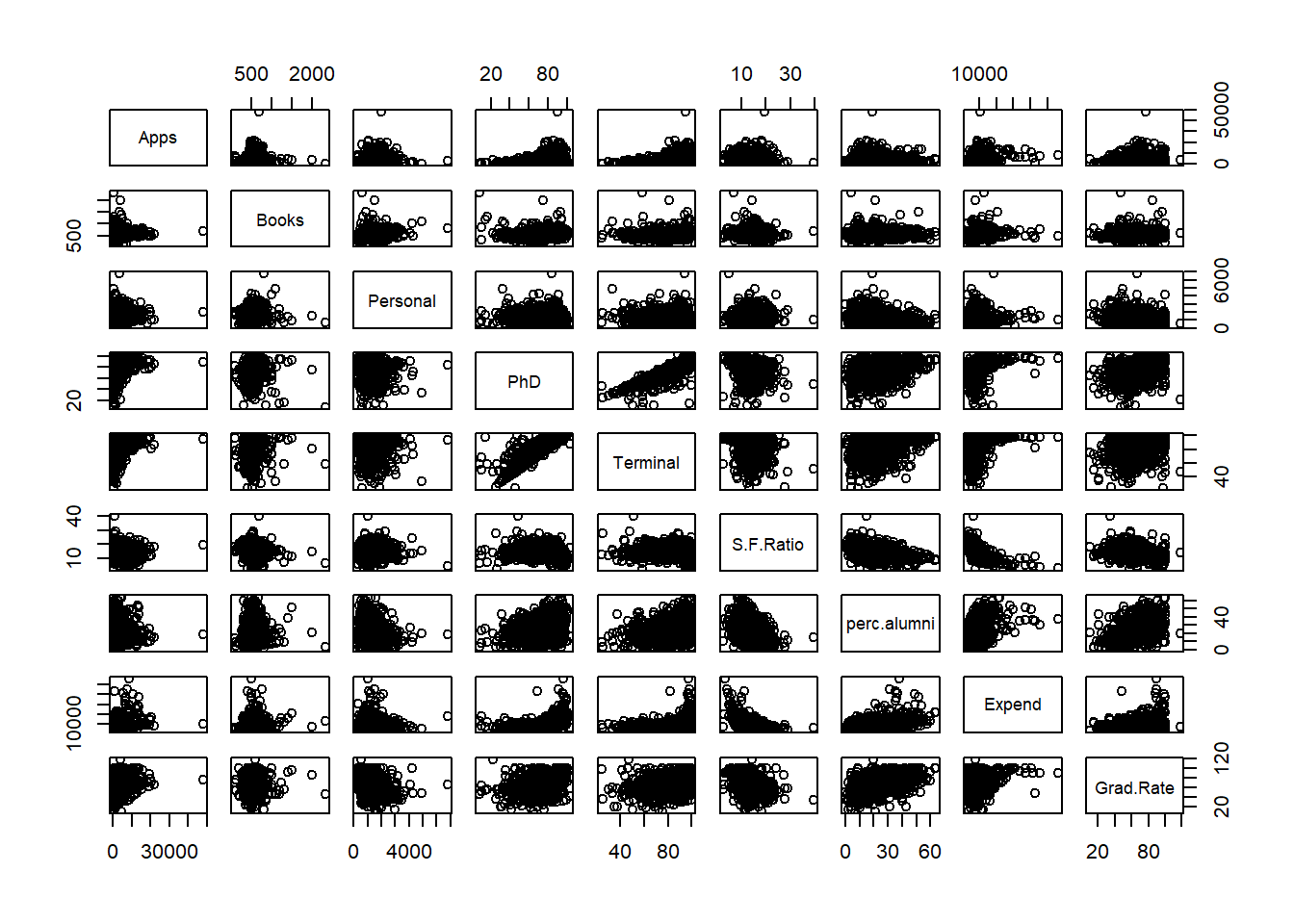In the second pairs plot there does not seem to be any strong relationships among the other variables.

Let us go a little deeper into the relationship between apps and enroll:

plot(College$Apps, College$Enroll)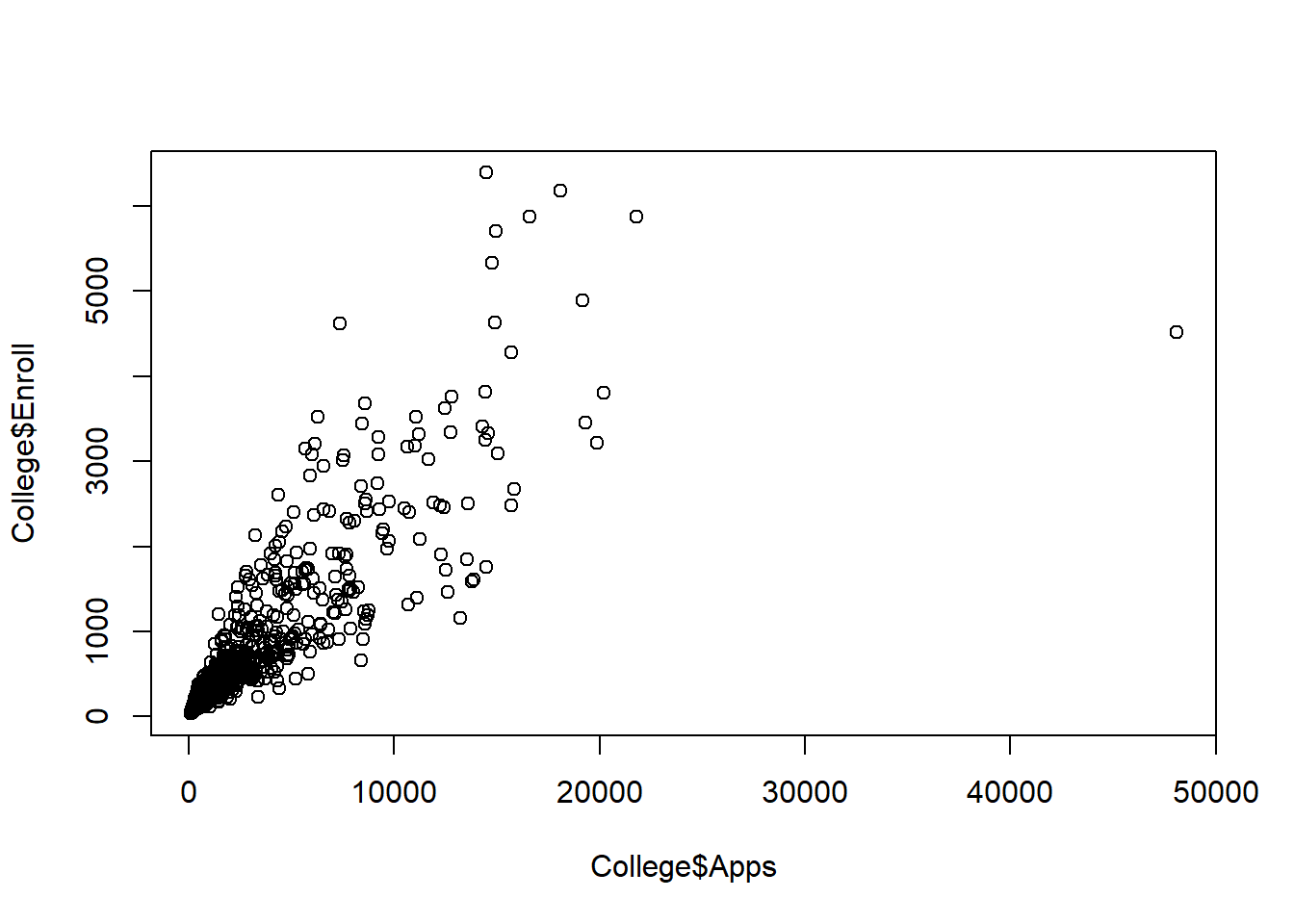Is there any relatioinship between the number of apps and whether a school is private or public?

plot(College$Private, College$Apps, xlab = "Private University", ylab = "# of Apps")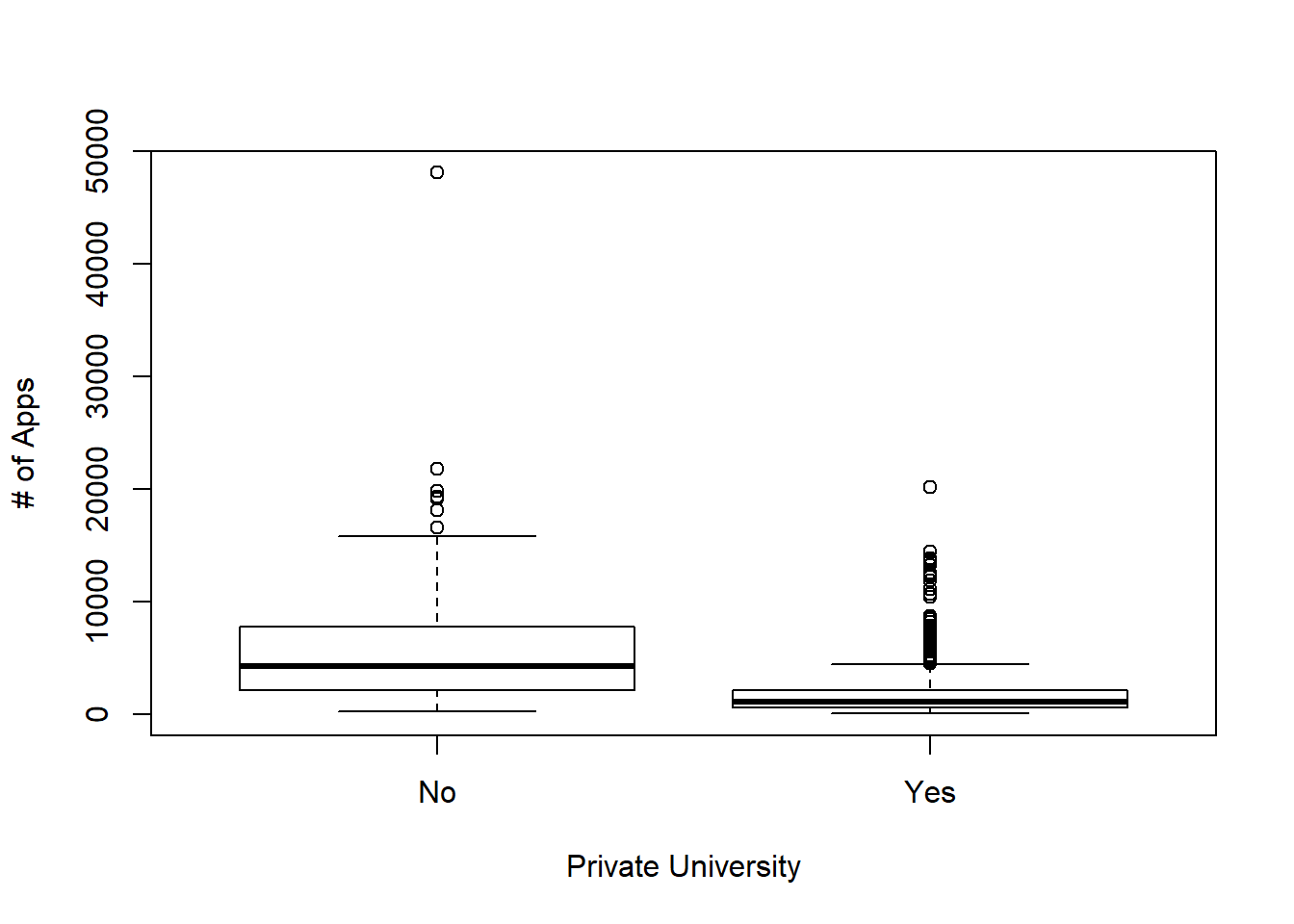We see that private colleges receive lower amounts of applications compared to public schools.

Now let us begin to build some prediction models. We will begin by splitting our data into training and test data.

set.seed(11)
train.size = dim(College) / 2
train = sample(1:dim(College), train.size)
test = -train
College.train = College[train, ]
College.test = College[test, ]

We have our test and train split, let us fit a least-squares regression model using best subsets onto the training set regressing apps onto all the variables:

library(leaps)
lm.subset.fit = regsubsets(Apps~., data = College.train)
reg.summary = summary(lm.subset.fit)
reg.summary
## Subset selection object
## Call: regsubsets.formula(Apps ~ ., data = College.train)
## 17 Variables  (and intercept)
##             Forced in Forced out
## PrivateYes      FALSE      FALSE
## Accept          FALSE      FALSE
## Enroll          FALSE      FALSE
## Top10perc       FALSE      FALSE
## Top25perc       FALSE      FALSE
## Outstate        FALSE      FALSE
## Room.Board      FALSE      FALSE
## Books           FALSE      FALSE
## Personal        FALSE      FALSE
## PhD             FALSE      FALSE
## Terminal        FALSE      FALSE
## S.F.Ratio       FALSE      FALSE
## perc.alumni     FALSE      FALSE
## Expend          FALSE      FALSE
## 1 subsets of each size up to 8
## Selection Algorithm: exhaustive
##          PrivateYes Accept Enroll Top10perc Top25perc F.Undergrad
## 1  ( 1 ) " "        "*"    " "    " "       " "       " "
## 2  ( 1 ) " "        "*"    " "    " "       " "       " "
## 3  ( 1 ) "*"        "*"    " "    " "       " "       " "
## 4  ( 1 ) " "        "*"    " "    "*"       " "       " "
## 5  ( 1 ) "*"        "*"    " "    "*"       " "       " "
## 6  ( 1 ) " "        "*"    "*"    "*"       " "       "*"
## 7  ( 1 ) " "        "*"    "*"    "*"       "*"       "*"
## 8  ( 1 ) " "        "*"    "*"    "*"       "*"       "*"
##          P.Undergrad Outstate Room.Board Books Personal PhD Terminal
## 1  ( 1 ) " "         " "      " "        " "   " "      " " " "
## 2  ( 1 ) " "         " "      " "        " "   " "      " " " "
## 3  ( 1 ) " "         " "      " "        " "   " "      " " " "
## 4  ( 1 ) " "         "*"      " "        " "   " "      " " " "
## 5  ( 1 ) " "         "*"      " "        " "   " "      " " " "
## 6  ( 1 ) " "         "*"      " "        " "   " "      " " " "
## 7  ( 1 ) " "         "*"      " "        " "   " "      " " " "
## 8  ( 1 ) " "         "*"      " "        " "   " "      " " " "
## 1  ( 1 ) " "       " "         " "    " "
## 2  ( 1 ) " "       " "         "*"    " "
## 3  ( 1 ) " "       " "         "*"    " "
## 4  ( 1 ) " "       " "         "*"    " "
## 5  ( 1 ) " "       " "         "*"    " "
## 6  ( 1 ) " "       " "         "*"    " "
## 7  ( 1 ) " "       " "         "*"    " "
## 8  ( 1 ) " "       " "         "*"    "*"

We will need to pick one of the models from the best subsets. Let us see what aspects of the modls we can pick.

names(reg.summary)
##  "which"  "rsq"    "rss"    "adjr2"  "cp"     "bic"    "outmat" "obj"

Looks like we have a few choices, let us decide out model by the adjusted r^2. Let us plot the adj r-squared and rss for the models.

reg.summary$rsq ##  0.8790639 0.9142662 0.9195393 0.9256525 0.9266580 0.9278003 0.9288231 ##  0.9295679 par(mfrow=c(2,2)) plot(reg.summary$rss,xlab="Number of Variables",ylab="RSS",type="l")
plot(reg.summary$adjr2,xlab="Number of Variables",ylab="Adjusted RSq",type="l")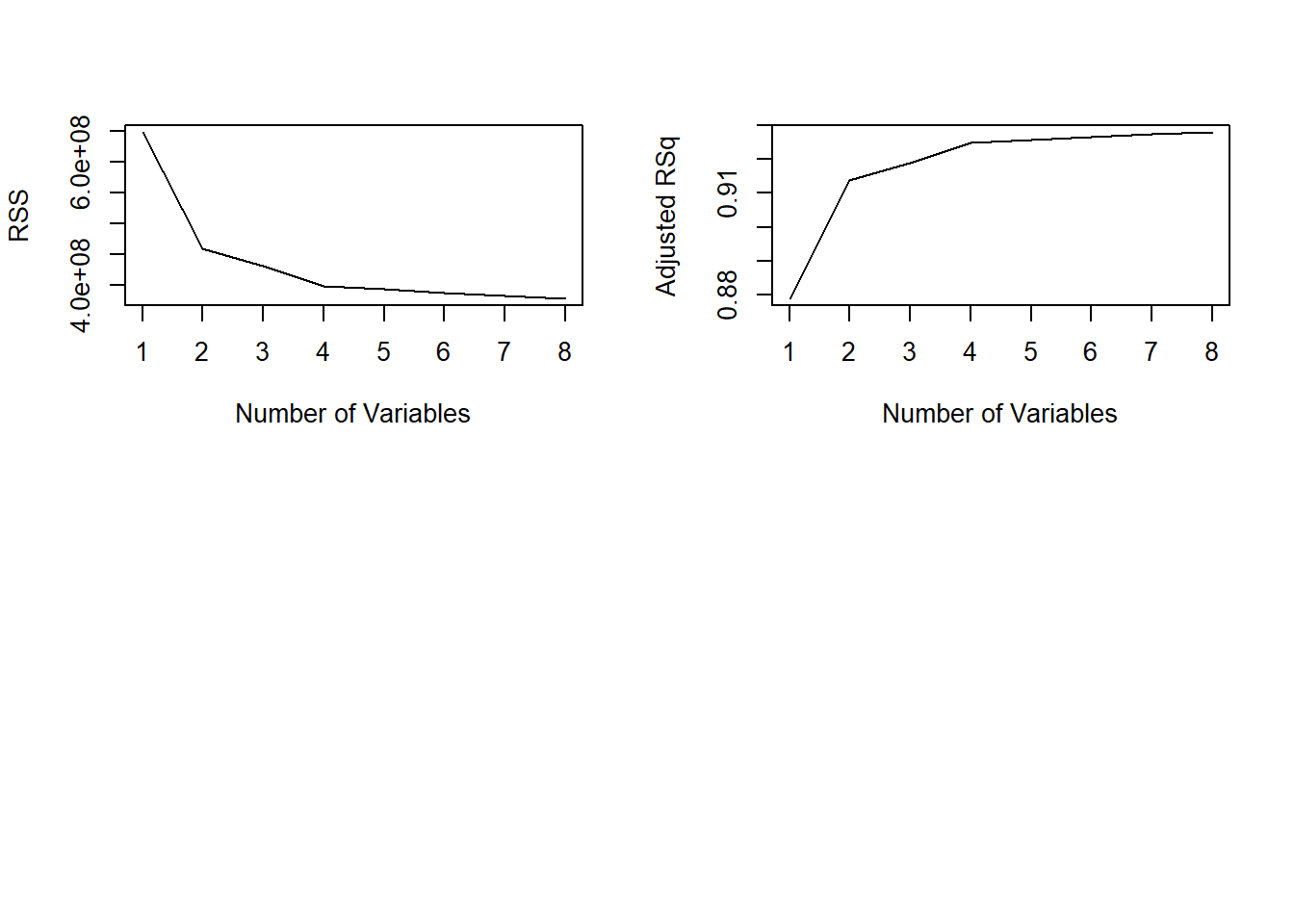Which one has the highest adj r-squared? which.max(reg.summary$adjr2)
##  8

Model number 8 has the highest r-squared. How about the lowest BIC.

which.min(reg.summary$bic) ##  4 Model 4 has the lowest BIC. Since BIC penalizes adding more variables, we will use Model 4 which contains Accept, top10percent, Outstate, and Expenditure. We will fit the OLS using these variables. lm.fit = lm(Apps~Accept + Top10perc + Outstate + Expend, data = College.train) lm.pred = predict(lm.fit, College.test) mean((College.test[, "Apps"] - lm.pred)^2) ##  1570988 The test error (RSS) is 1570988. Now let us see how well a ridge regression model will work. We will need to load the glmnet library. library(glmnet) ## Loading required package: Matrix ## Loading required package: foreach ## Loaded glmnet 2.0-16 We will fit the ridge regression to the training data and also will pick lambda based on cross-validation: train.mat = model.matrix(Apps~., data=College.train) test.mat = model.matrix(Apps~., data=College.test) grid = 10 ^ seq(4, -2, length=100) mod.ridge = cv.glmnet(train.mat, College.train[, "Apps"], alpha=0, lambda=grid, thresh=1e-12) lambda.best = mod.ridge$lambda.min
lambda.best
##  18.73817

The best lambda is 18.74 or ~19. Now we will run the model with the best lambda.

ridge.pred = predict(mod.ridge, newx=test.mat, s=lambda.best)
mean((College.test[, "Apps"] - ridge.pred)^2)
##  1608859

Our test error (RSS) is 1608859, which is a little higher than the OLS model we fit before.
Lasso regression should bring some our our coefficients to zero. Let us fit the Lasso regression to the training set.

mod.lasso = cv.glmnet(train.mat, College.train[, "Apps"], alpha=1, lambda=grid, thresh=1e-12)
lambda.best = mod.lasso$lambda.min lambda.best ##  21.54435 The best lambda is 21.544 or rounded to 22. Now let us predict using this lambda. lasso.pred = predict(mod.lasso, newx=test.mat, s=lambda.best) mean((College.test[, "Apps"] - lasso.pred)^2) ##  1635280 Our test error is 1635280 which is higher than our Ridge and OLS models. Let us take a look at the coefficients: mod.lasso = glmnet(model.matrix(Apps~., data=College), College[, "Apps"], alpha=1) lasso.coef = predict(mod.lasso, s=lambda.best, type="coefficients") lasso.coef ## 19 x 1 sparse Matrix of class "dgCMatrix" ## 1 ## (Intercept) -6.038452e+02 ## (Intercept) . ## PrivateYes -4.235413e+02 ## Accept 1.455236e+00 ## Enroll -2.003696e-01 ## Top10perc 3.367640e+01 ## Top25perc -2.403036e+00 ## F.Undergrad . ## P.Undergrad 2.086035e-02 ## Outstate -5.781855e-02 ## Room.Board 1.246462e-01 ## Books . ## Personal 1.832910e-05 ## PhD -5.601313e+00 ## Terminal -3.313824e+00 ## S.F.Ratio 4.478684e+00 ## perc.alumni -9.796600e-01 ## Expend 6.967693e-02 ## Grad.Rate 5.159652e+00 How many are non-zero coefficients are there? lasso.coef[lasso.coef!=0] ## <sparse>[ <logic> ] : .M.sub.i.logical() maybe inefficient ##  -6.038452e+02 -4.235413e+02 1.455236e+00 -2.003696e-01 3.367640e+01 ##  -2.403036e+00 2.086035e-02 -5.781855e-02 1.246462e-01 1.832910e-05 ##  -5.601313e+00 -3.313824e+00 4.478684e+00 -9.796600e-01 6.967693e-02 ##  5.159652e+00 There are 16 variables that are non-zero. Let us fit a PCR model: library(pls) ## ## Attaching package: 'pls' ## The following object is masked from 'package:stats': ## ## loadings pcr.fit = pcr(Apps~., data=College.train, scale=T, validation="CV") validationplot(pcr.fit, val.type="MSEP")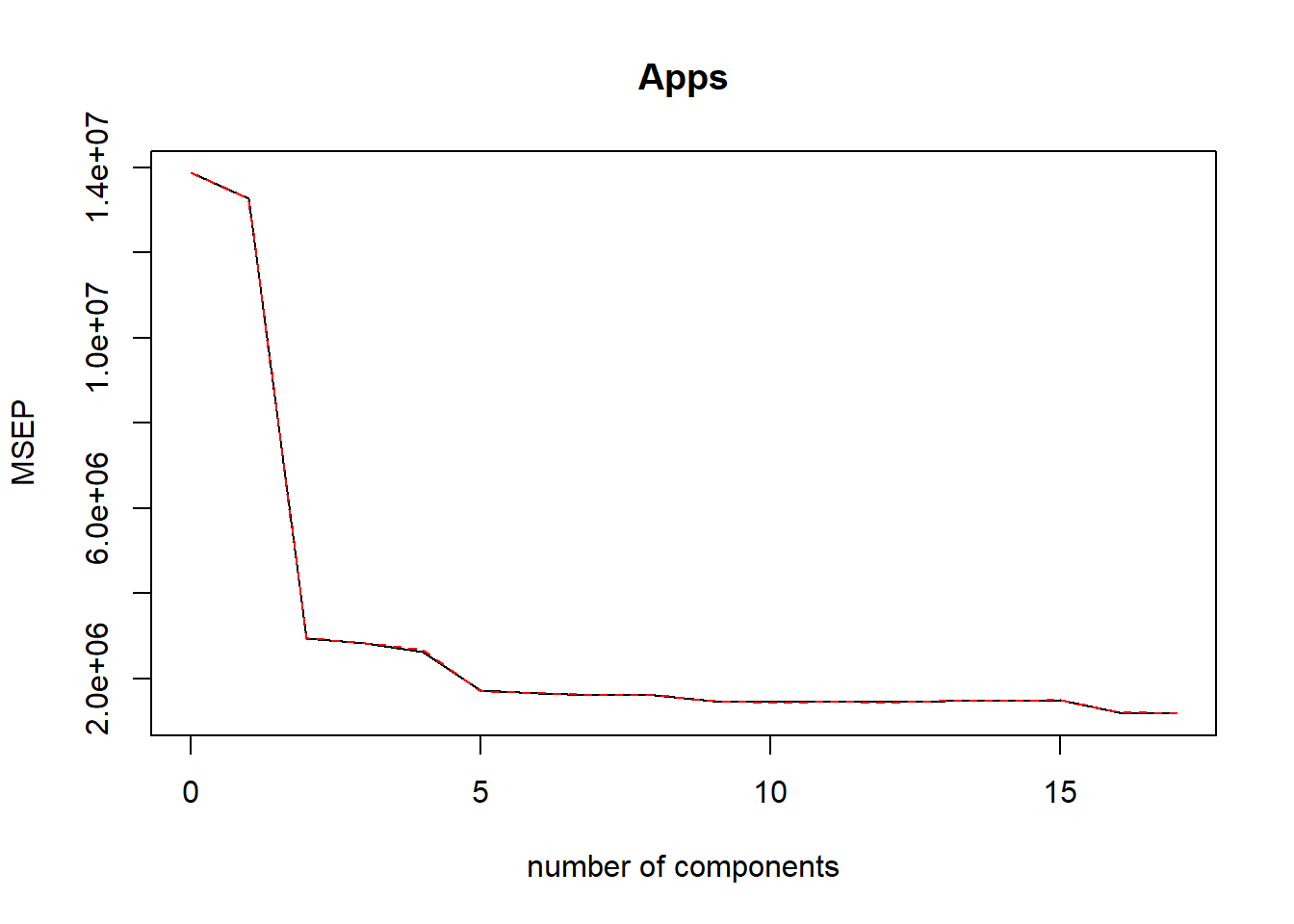Prediction using PCR and with the number of components at 10. pcr.pred = predict(pcr.fit, College.test, ncomp=10) mean((College.test$Apps - pcr.pred)^2)
##  3014496

The test error for PCR is quite high at 3014496.

Now let us perform a PLS fit to the data and see how well it fits.

pls.fit = plsr(Apps~., data=College.train, scale=T, validation="CV")
validationplot(pls.fit, val.type="MSEP")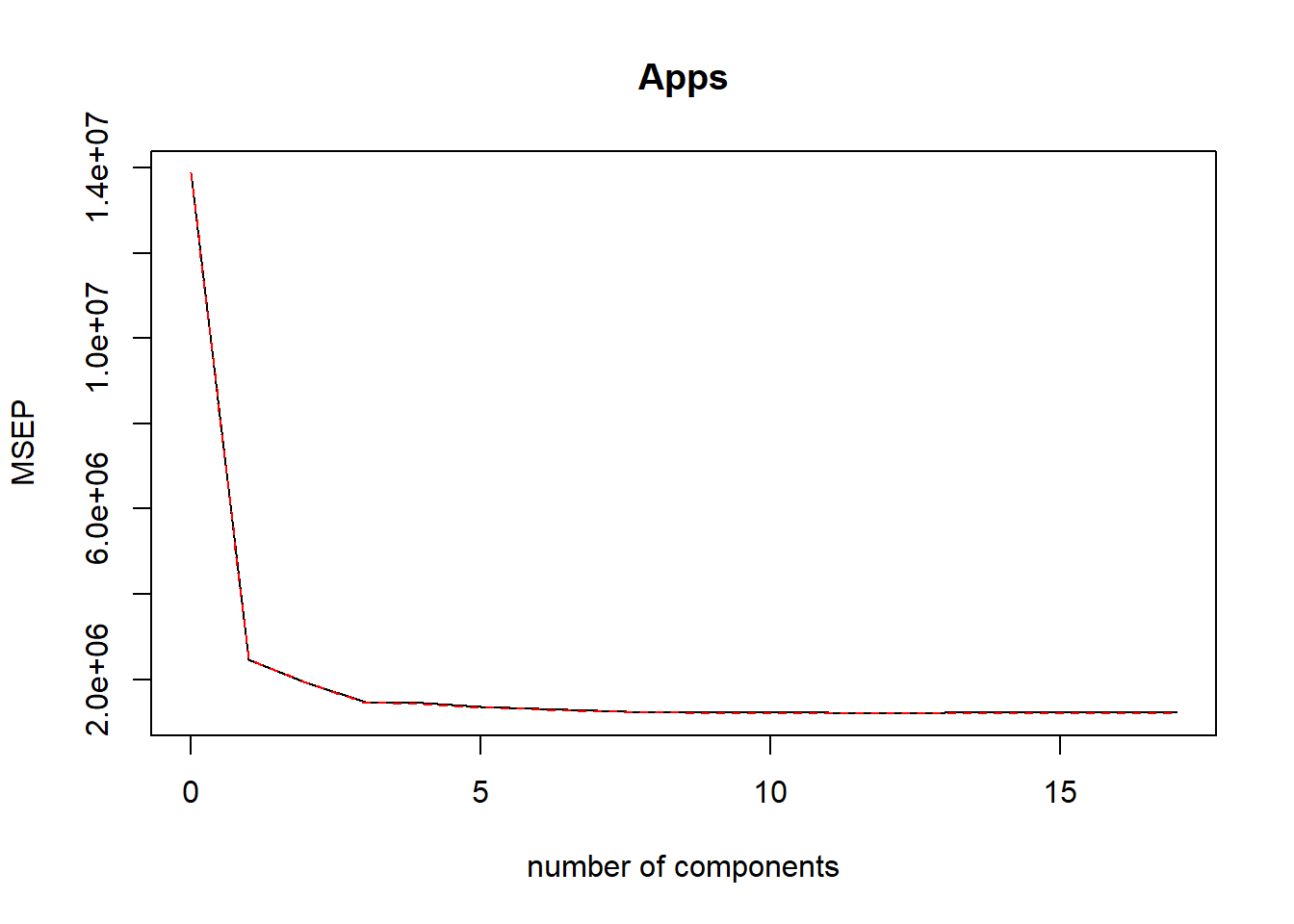We use the same number of components at 10. How does this model fair?

pls.pred = predict(pls.fit, College.test, ncomp=10)
mean((College.test\$Apps - pls.pred)^2)
##  1508987

Our Test error is 1508987 which is better than all of our models so far.

## Conclusion

After test a variety of models fit using various methods such as: best subsets, ridge, lasso, PCR, and PLS. We found that the performance from best to worst was:
1. PLS with a RSS of 1508987
2. Best Subset (using model number 4) with a RSS of 1570988.
3. Ridge with a RSS of 1608859. 4. Lasso with a RSS of 1635280. 5. PCR with a RSS of 3014496.

All in all, we see that PLS performed the best out of the 5 different methods. This is not always the case when fitting regression models as the best performing model can vary depending on the data and objective of your model. I believe PLS performed the best because it was able to standardize the variables and breakdown the variation into separate pieces and then fitting the standardize variables using OLS. Keep in mind though that PLS is not always the most accurate or best performing model.